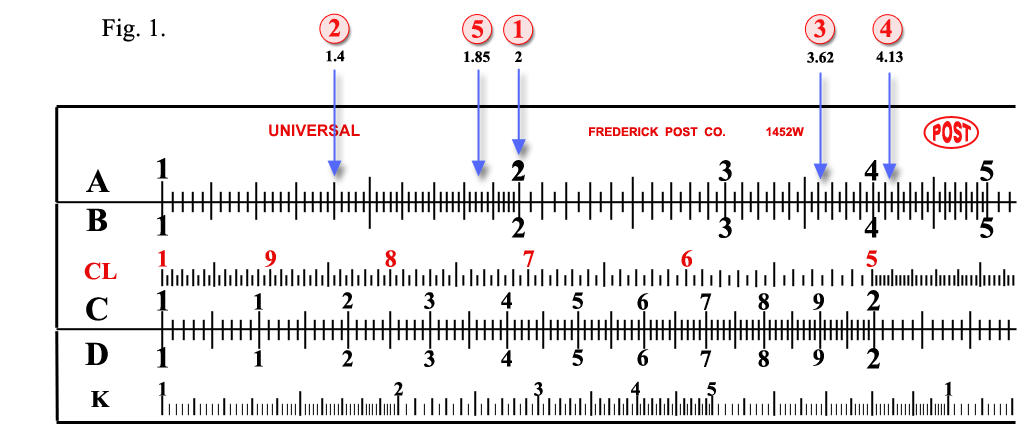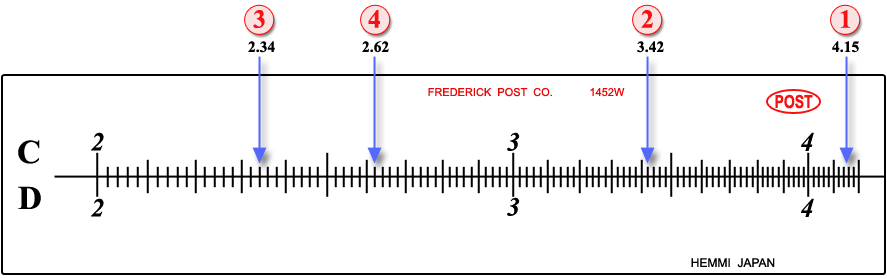The Scales.On the face of the slide rule you see four scales A, B, C and D and on the back face of the slide, you see three scales S, L and T. Of these scales A, B, C and D are for multiplication, division, squaring, extraction of a square root, cubing and extraction of a cube root, T and S are for trigonometrical functions, a sine and a tangent respectively, and L for logarithms. Naturally on the slide rules for beginners the scales on the back face, S, L and T are often destroyed.The greatest importance in using a slide rule is the reading. The accuracy in reading means the accuracy in calculation. Hence the practice of a slide rule is the practice of its reading.Of these scales A and B are exactly the same and so are C and D. To begin with A and B, you will read 1 at the left end of the scale, and then gradually 2, 3, 4......to the right. At the center, you read 10; then to the right you will read 20, 30, 40......until you come to 100 at the right end.
 The sub-divisions represent each, either 1/10 or 1/5 or 1/2 of the sub-division just like those on any rule of the decimal system. The only trouble for beginners would be the un-equality or variations of divisions; but they will overcome it after a little practice.Repeating the explanation, read first the large division, then the sub-division and then the lesser sub-division; these three figures put together in order represent the digit value of the point on the scale. A point between lines, or a point that is not marked, is to be read by inspection.For examples five points, (1), (2), (3)......, (5) are taken on A, B and four points, (1), (2)......, (4) are taken on C, D scales (see, Fig. 1): (1) on A, B, is to read “2,” (2) “1.4,” (3) “3.62,” (4) “4.13,” (5) “1.85”. Furthermore (1), (2), (3), (4)......on C, D represent 4.15, 3.42, 2.34, 2.62,......respectively.Note the divisions on C and D scales of a 5″ slide rule are exactly the same as those on the left half section of A and B of a 10″ slide rule.